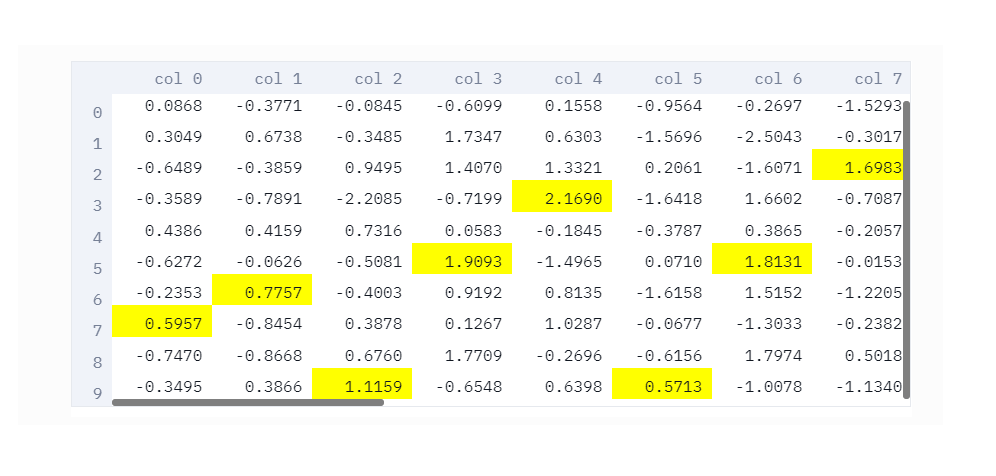# st.dataframe - 显示可交互数据帧

## 方法原型

``````streamlit.dataframe(data=None, width=None, height=None)
``````

• data：要显示的数据对象，类型可以是pandas.DataFrame、pandas.Styler、numpy.ndarray、Iterable、dict或None。 如果是pandas.Styler对象，那么将被应用到DataFrame对象上。Streamlit支持自定义单元值和颜色，不过不支持 pandas的某些样式特性，例如条形图、鼠标悬停效果和说明文字。Styler的支持目前还是实验性质的。
• width：UI元素的期望宽度，单位：像素，类型为Int或None，如果是None的话，Streamlit将基于页面宽度计算元素宽度
• height：UI元素的期望高度，单位：像素，类型为Int或None

## 调用代码

``````>>> df = pd.DataFrame(
...    np.random.randn(50, 20),
...    columns=('col %d' % i for i in range(20)))
...
>>> st.dataframe(df)  # Same as st.write(df)
````````````>>> st.dataframe(df, 200, 100)
``````

``````>>> df = pd.DataFrame(
...    np.random.randn(10, 20),
...    columns=('col %d' % i for i in range(20)))
...
>>> st.dataframe(df.style.highlight_max(axis=0))
``````